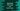# What is union type in typescript and how to use## What is union type in typescript and how to use:

Union is a way in typescript to define a variable that can hold multiple types of values. For example, we can create a variable that can hold both string and int type. In this post, we will learn how to use union type in typescript with example.

### Syntax of union type:

Pipe symbol (|) is used for union type. Below is the syntax of union type:

``let varName: type1 | type2``

### Example of union type:

Let’s take a look at the below example:

``````let myVariable : string | boolean;

myVariable = 'Hello';
myVariable = true;

myVariable = 20;``````

In this example, we created one variable myVariable of type string | boolean. We are assigning three different types of data to this variable.

In the last line, we are assigning one number to this variable myVariable. It will throw one compile-time error:

``type '20' is not assignable to type 'string | boolean'``

There is no limit of types we can add to union type. For example, if we add number as another type, this error will be removed:

``````let myVariable : string | boolean | number;

myVariable = 'Hello';
myVariable = true;

myVariable = 20;``````